Accelerate your child’s learning
Smartick is a fun way to learn math!Dec28

# Division Exercises: Learning the Concept of Division

In this post, we are going to check out some examples of division exercises in order to learn the concept of division.

Dividing is splitting something up into equal parts and in order to understand it, let’s look at this concept with the help of some exercises to understand all the steps of division, as well as to see what is a remainder of division.

## Division exercises number one: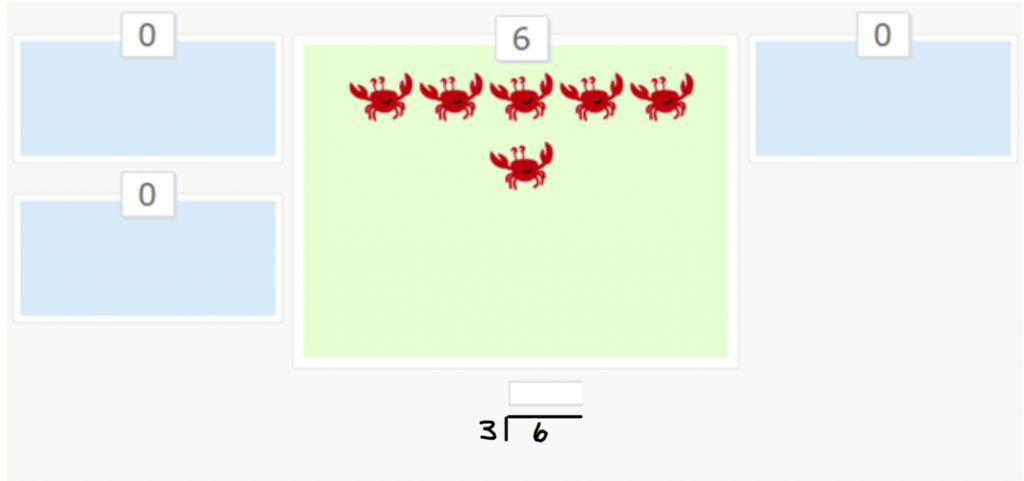In this example, we have to divide the 6 crabs into 3 boxes equally. If we divide the crabs equally into the boxes, we’ll have 2 crabs in each box.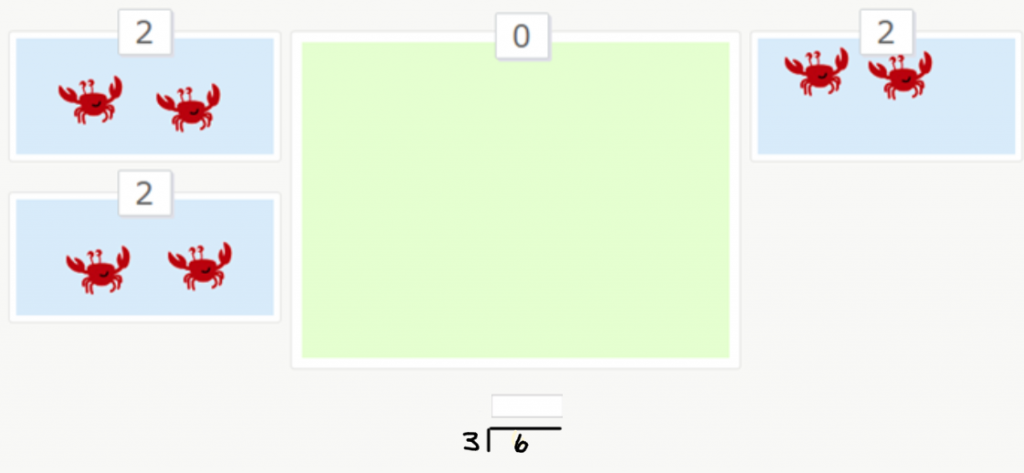This means that we are dividing 6 by 3 and our answer is 2. In the next picture, in the lower part of the image, you can see the way the division problem is written: the number that we want to divide by is written on the left side of the box, the number that we are dividing is written inside of the box and the answer is written above the box: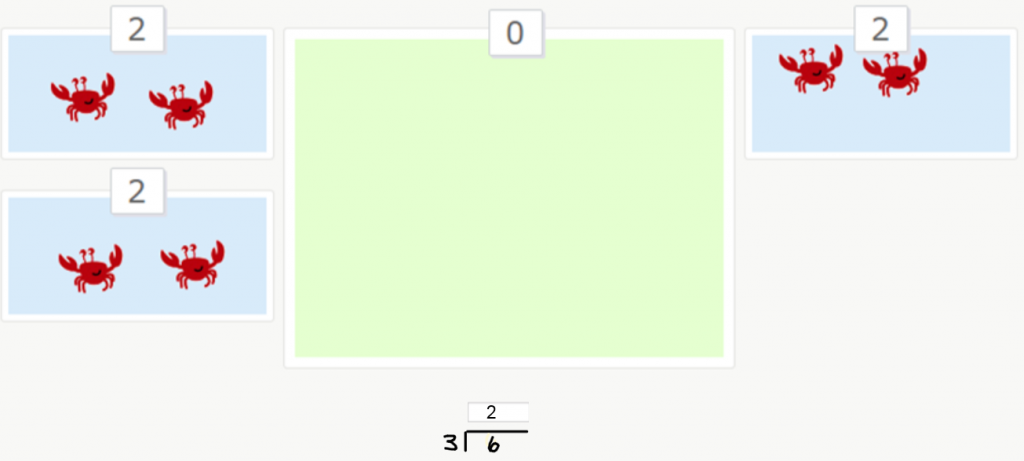Now, let’s take a look at another example of division problems with remainders.

## Division exercises number two: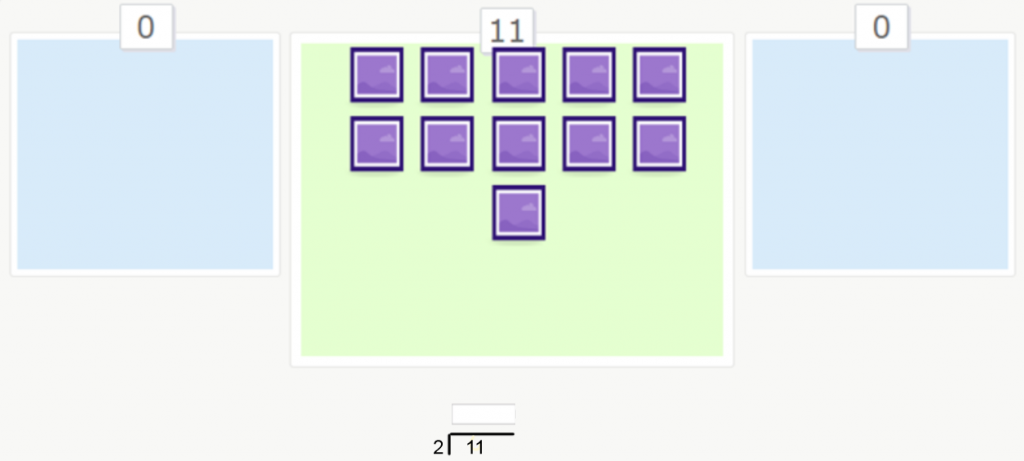In this example, we have to divide 11 squares into 2 groups, and we have to do it so each group has an equal amount of squares. If we divide the squares into equal groups, we get two groups of 5 squares. There is 1 square leftover and it is the remainder of the division problem: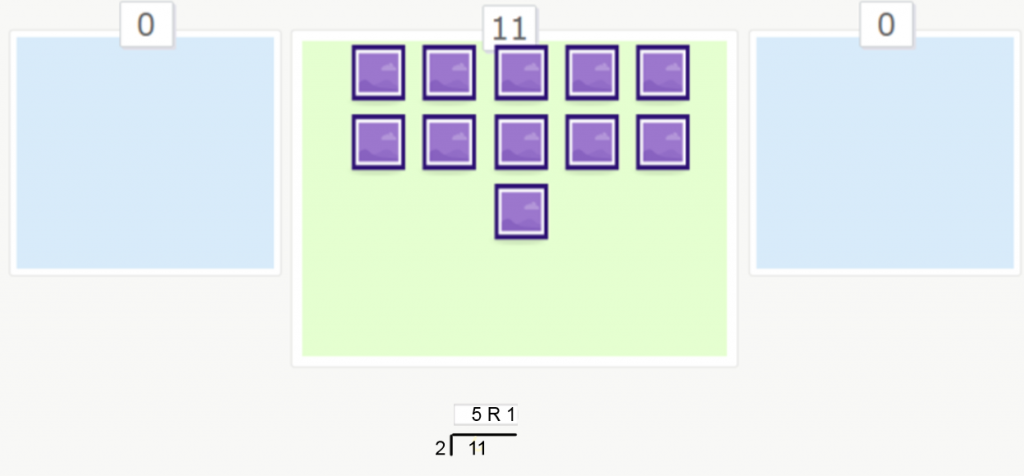I’m sure that these examples have helped you understand division. In other posts, we’ll put up different examples in order to see the process of solving a longer division problem, for example, dividing by 1, 2 or 3 digit numbers.

If you liked this post, share it with your friends and classmates so that they can learn how to solve division problems with and without remainders. And, if you want to keep on practicing division or any other elementary math topic, you can create an account on Smartick and try it for free.